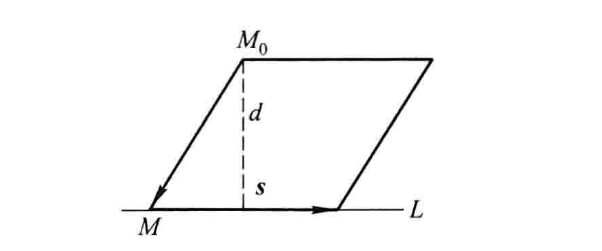• 高等代数与解析几何,高等代数与解析几何,高等代数与解析几何,高等代数与解析几何.
• 高等代数与解析几何课程可视化教学探析，余柏林，王冬冬，本文中，通过对高等代数与解析几何课程教学现状的调查和分析，提出了加强本课程中抽象概念教学的三个方法和运用计算机代数系统Map
• 高等代数与解析几何(下)(第2版)》是《高等代数与解析几何》的修订版，主要有两大基本特色，一是把几何的观念和代数的方法结合起来组织教学，二是引入相关数学软件来实践代数几何中的一些基本问题，并提供网上...
• 高等代数与解析几何-孟道骥 上册 线性代数与解析几何结合讲解
• 陈志杰 高等代数与解析几何答案 第一章 向量代数 习题解答
• 高等代数与解析几何 高教版 第一章 课本详细答案
• 由陈志杰主编，高等教育出版社发行的《高等代数与解析几何》的课后习题配套解答
• 高等代数与解析几何。高等教育出版社。作者：孟道骥
• 本书包含了原高等代数与解析几何两门基础课的主要内容的答案。　（习题答案）
• 这里的是高等代数与解析几何的答案 由陈志杰编写的那本书的。。。希望对大家有帮助！
• 1.$(15')$ $x_1=x_2=1,x_n=x_{n-1}+x_{n-...则空间中所有\overrightarrow{n}垂直的平面F交线的对称中心在一条直线上 转自: http://www.math.org.cn/forum.php?mod=viewthread&tid=37137  1.(15') \[x_1=x_2=1,x_n=x_{n-1}+x_{n-2}.$
试用矩阵论方法给出$x_n$通项.
2.$(15')$
$\alpha,\beta$为欧氏空间$V$中两个长度相等的向量.证明存在正交变换$A$使得$A\alpha=\beta$
3.$(10')$证明$n$阶$\mathrm{Hermite}$矩阵$A$有$n$个实特征值(考虑重数).
4.$(20')$.$F$为数域
$\alpha_1,\alpha_2\cdots \alpha_n,\beta_1,\beta_2,\cdots \beta_n$
是$F^n$中$2n$个列向量.
用$\left|\alpha_1,\cdots \alpha_n\right|$表示以$\alpha_1,\alpha_2\cdots \alpha_n$为列向量的矩阵的行列式.证明下面的行列式等式
$\left|\alpha_1,\cdots \alpha_n\right|\cdot \left|\beta_1,\cdots \beta_n\right|=\sum_{i=1}^n \left|\alpha_1,\cdots \alpha_{i-1},\beta_1,\alpha_{i+1},\cdots \alpha_n\right|\cdot \left|\alpha_i, \beta_2,\cdots \beta_n\right|$
5.$(20')$
$F$为数域,$V$是$F$上$n$维线性空间.$A$是$V$上线性变换.证明存在唯一可对角化线性变换$A_1$,幂零线性变换$A_2$
使得
$A=A_1+A_2,A_1A_2=A_2A_1$
6.$(20')$
$F$为数域,$A,B,P\in M_n(F)$,$P$幂零且
$(A-B)P=P(A-B),BP-PB=2(A-B)$
求一个可逆矩阵$Q$使得$AQ=QB$.
7.$(15')$. $\vec{a},\vec{b},\vec{c}$共面的充要条件为$\vec{a}\times \vec{b},\vec{b}\times \vec{c},\vec{c}\times \vec{a}$共面
8.$(20')$空间中四点$O,A,B,C$使得
$\angle AOB=\frac{\pi}{2},\angle BOC=\frac{\pi}{3},\angle COA=\frac{\pi}{4}$
设$AOB$决定的平面为$\pi_1$,$BOC$决定的平面为$\pi_2$,求$\pi_1,\pi_2$二面角.求出二面角的余弦值即可.
9.$(15')$
$F$为单叶双曲面,$\overrightarrow{n}$为给定非零向量.
则空间中所有与$\overrightarrow{n}$垂直的平面与$F$交线的对称中心在一条直线上


展开全文• 9. $(15')$ 平面 $Ax+By+Cz+D=0$ 双曲抛物面 $2z=x^2-y^2$ 交于两条直线. 证明 $A^2-B^2-2CD=0$.    10. $(15')$ 正十二面体有 $12$ 个面, 每个面为正五边形, 每个顶点连接 $3$ 条棱. 求它的内切球外接球...
本文来自TangSong.

1. $(10')$ 在 $\bbR^3$ 上定义线性变换 $\scrA,\ \scrA$ 在自然基 $\varepsilon_1=\left(\begin{array}{c} 1\\ 0\\ 0\end{array}\right),\varepsilon_2=\left(\begin{array}{c} 0\\ 1\\ 0\end{array}\right),\varepsilon_3=\left(\begin{array}{c} 0\\ 0\\ 1\end{array}\right)$ 下的矩阵为 $\left(\begin{array}{ccc} 0&1&-1\\ 0&0&1\\ 0&0&0\end{array}\right)$ 求 $\bbR^3$ 的一组基, 使得 $\scrA$ 在这组基下具有 Jordan 型.

2. $(10')$ $3$ 阶实矩阵 $A$ 的特征多项式为 $x^3-3x^2+4x-2$. 证明$A$ 不是对称阵也不是正交阵.

3. $(15')$ 在所有 $2$ 阶实方阵上定义二次型 $f$:$X\rightarrow \tr(X^2)$, 求 $f$ 的秩和符号差.

4. $(15')$ 设 $V$ 是有限维线性空间, $\scrA,\scrB$ 是 $V$ 上线性变换满足下面条件:
(1) $\scrA\scrB=\scrO$;
(2) $\scrA$ 的任意不变子空间也是 $\scrB$ 的不变子空间;
(3) $\scrA^5+\scrA^4+\scrA^3+\scrA^2+\scrA=\scrO$.
证明 $\scrB\scrA=\scrO$.

5. $(15')$ 设 $V$ 是全体次数不超过 $n$ 的实系数多项式组成的线性空间. 定义线性变换$\scrA$:$f(x)\rightarrow f(1-x)$. 求 $\scrA$ 的特征值和对应的特征子空间.

6. $(15')$ 计算行列式.各行底数为等差数列,各列底数也为等差数列,所有指数都是$50$: $\left|\begin{array}{ccccc} 1^{50}&2^{50}&3^{50}&\cdots &100^{50}\\ 2^{50}&3^{50}&4^{50}&\cdots &101^{50}\\ \vdots&\vdots&\vdots&\ddots&\vdots\\ 100^{50}&101^{50}&102^{50}&\cdots& 199^{50}\\\end{array}\right|.$

7. $(20')$ 设 $V$ 是复数域上有限维线性空间, $\scrA$ 是 $V$ 上可线性变换, $\scrA$ 在一组基下矩阵为 $F$. 证明:
(1) 若 $\scrA$ 可对角化, 对任意 $\scrA$ 的不变子空间 $U$, 存在$U$ 的一个补空间 $W$ 是 $\scrA$ 的不变子空间;
(2) 若对任意 $\scrA$ 的不变子空间 $U$, 存在 $U$ 的一个补空间 $W$ 是 $\scrA$ 的不变子空间,证明 $F$ 可对角化.

8. $(20')$ 平面上一个可逆仿射变换将一个圆映为椭圆或圆. 详细论证这一点.

9. $(15')$ 平面 $Ax+By+Cz+D=0$ 与双曲抛物面 $2z=x^2-y^2$ 交于两条直线. 证明 $A^2-B^2-2CD=0$.

10. $(15')$ 正十二面体有 $12$ 个面, 每个面为正五边形, 每个顶点连接 $3$ 条棱. 求它的内切球与外接球半径比. 
展开全文• 第四讲应用MATLAB解决高等代数问题;例求解线性方程组;经过初等行变换将矩阵;A=[3 -1 5 3;1 -1 2 1;1 -2 -1 2] %输入矩阵的数据 A([1 3])=A([3 1]) %交换第一行和第三行数据 A(2)=A(2)-A(1) %将第一行乘以-1加到第二...
• 这是一个高等代数与空间解析几何部分选讲课件，专为数学专业的同学设计！也可作为考研辅导题目！是我们老师的心血，也不知道他会骂我不！不管了，先放上去了！希望更多的人喜欢数学！数学竞赛
• “线性代数与空间解析几何”是研究有限维空间的线性结构线性理论及其几何背景的课程。通过本课程学习，使学生不仅要掌握线性代数的基本概念和基本理论，而且要掌握代数中提出问题、分析问题和解决问题的思想方法，...
• 作者: 潘晏仲 出版社: 西安交通大学出版社 译者: 李洪军 出版年: 1999年10月1日 页数: 494 页 ...　本书可作为理工科院校数学类各专业的高等代数及高等几何（含空间解析几何）教材或教学参考书。
• 李永乐复习全书高等数学 第四章 向量代数与空间解析几何 易错题和难题记录
目录4.2  平面与直线例9  求过直线$L:\cfrac{x-1}{2}=\cfrac{y+2}{-3}=\cfrac{z-2}{2}$且垂直于平面$\varPi:3x+2y-z-5=0$的平面方程。例12  直线$L_1:\cfrac{x-1}{1}=\cfrac{y+1}{2}=\cfrac{z-1}{\lambda}$和直线$L_2:x+1=y-1=z$是否相交？如果相交求其交点，如果不相交求两直线间的距离。例14  空间$n$个点$P_i(x_i,y_i,z_i)(i=1,2,\cdots,n;n\geqslant4)$，矩阵$\bm{A}=\begin{bmatrix}x_1&y_1&z_1&1\\x_2&y_2&z_2&1\\\vdots&\vdots&\vdots&\vdots\\x_n&y_n&z_n&1\\\end{bmatrix}$的秩记为$r$，则$n$个点共面的充分必要条件是（  ）$(A)r=1;$$(B)r=2;$$(C)r=3;$$(D)1\leqslant r\leqslant3.$4.3  空间曲面与曲线例16  求以曲线$\varGamma:\begin{cases}x^2+y^2+z^2=1,\\x+y+z=0\end{cases}$为准线，母线平行于直线$x=y=z$的柱面方程。例19  求直线$L:\cfrac{x-1}{1}=\cfrac{y}{1}=\cfrac{z-1}{-1}$在平面$\varPi:x-2y+2z-1=0$上的投影直线$l_0$的方程，并求$l_0$绕$y$旋转一周所成的曲线方程。例21  求曲线$L:\begin{cases}x^2+y^2+z^2=a^2(a>0)\\x^2+y^2=ax\end{cases}$分别在$xOy$面和$xOz$面上投影曲线方程。练习四9.求曲线$L:\begin{cases}x=t,\\y=t^2,\\z=e^t,\end{cases}$绕$z$轴旋转一周所得旋转曲面的方程。写在最后
4.2  平面与直线
例9  求过直线$L:\cfrac{x-1}{2}=\cfrac{y+2}{-3}=\cfrac{z-2}{2}$且垂直于平面$\varPi:3x+2y-z-5=0$的平面方程。
解  直线$L$的方向向量为$\bm{s}=(2,-3,2)$，平面$\varPi$的法向量为$\bm{n}_1=(3,2,-1)$，则所求平面的法向量为
$\bm{n}=\bm{s}\times\bm{n}_1=\begin{vmatrix}\bm{i}&\bm{j}&\bm{k}\\2&-3&2\\3&2&-1\end{vmatrix}=-\bm{i}+8\bm{j}+13\bm{k}.$
所求平面方程为$-(x-1)+8(y+2)+13(z-2)=0$，即$x-8y-13z+9=0$。（这道题主要利用了向量叉乘求解）
例12  直线$L_1:\cfrac{x-1}{1}=\cfrac{y+1}{2}=\cfrac{z-1}{\lambda}$和直线$L_2:x+1=y-1=z$是否相交？如果相交求其交点，如果不相交求两直线间的距离。
解  直线$L_1$的方向向量$\bm{s}_1=(1,2,\lambda)$，直线$L_2$的方向向量为$\bm{s}_2=(1,1,1)$。
$A(1,-1,1)$为$L_1$上的点，$B(-1,1,0)$为直线$L_2$上的点，则$\overrightarrow{AB}=(-2,2,-1)$。
直线$L_1$与$L_2$共面的充要条件是向量$\bm{s}_1,\bm{s}_2,\overrightarrow{AB}$混合积为零，即
$\begin{vmatrix} 1&2&\lambda\\ 1&1&1\\ -2&2&-1 \end{vmatrix}=0\Rightarrow\lambda=\cfrac{5}{4}.$
故当$\lambda=\cfrac{5}{4}$时，直线$L_1$与$L_2$相交，当$\lambda\ne\cfrac{5}{4}$时，$L_1$与$L_2$不相交。
当$\lambda=\cfrac{5}{4}$时，为求得直线$L_1$与$L_2$的交点，令$L_1:\cfrac{x-1}{1}=\cfrac{y+1}{2}=\cfrac{z-1}{\cfrac{5}{4}}=t$，则$x=1+t,y=-1+2t,z=1+\cfrac{5}{4}t$。代入$x+1=y-1=z$得$t=4$。则$L_1$与$L_2$交点为$(5,7,6)$。
当$\lambda\ne\cfrac{5}{4}$时，为求得直线$L_1$与$L_2$之间距离，考虑直线$L_1$和$L_2$的方向向量$\bm{s}_1=(1,2,\lambda),\bm{s}_2=(1,1,1)$和向量$\overrightarrow{AB}=(-2,2,-1)$，由两直线之间的距离公式知
$d=\cfrac{|(\bm{s}_1\bm{s}_2\overrightarrow{AB})|}{|\bm{s}_1\times\bm{s}_2|}=\cfrac{|4\lambda-5|}{\sqrt{(2-\lambda)^2+(\lambda-1)^2+1}}.$
（这道题主要利用了空间共面条件求解）
例14  空间$n$个点$P_i(x_i,y_i,z_i)(i=1,2,\cdots,n;n\geqslant4)$，矩阵$\bm{A}=\begin{bmatrix}x_1&y_1&z_1&1\\x_2&y_2&z_2&1\\\vdots&\vdots&\vdots&\vdots\\x_n&y_n&z_n&1\\\end{bmatrix}$的秩记为$r$，则$n$个点共面的充分必要条件是（  ）$(A)r=1;$$(B)r=2;$$(C)r=3;$$(D)1\leqslant r\leqslant3.$
解  设$n$个点共面，则其中任取$4$个点，例如$P_1,P_2,P_3$与$P_4$也必共面。于是
\begin{aligned} \begin{vmatrix}x_1&y_1&z_1&1\\x_2&y_2&z_2&1\\x_3&y_3&z_3&1\\x_4&y_4&z_4&1\end{vmatrix}&=\begin{vmatrix}x_1&y_1&z_1&1\\x_2-x_1&y_2-y_1&z_2-z_1&0\\x_3-x_1&y_3-y_1&z_3-z_1&0\\x_4-x_1&y_4-y_1&z_4-z_1&0\end{vmatrix}\\ &=\begin{vmatrix}x_2-x_1&y_2-y_1&z_2-z_1\\x_3-x_1&y_3-y_1&z_3-z_1\\x_4-x_1&y_4-y_1&z_4-z_1\end{vmatrix}=0, \end{aligned}
其中最后一个行列式为零来自三点式平面方程，所以$1\leqslant r\leqslant3$。反之，设$1\leqslant r\leqslant3$，则$\bm{A}$中任取一个$4$阶矩阵，其对应的行列式必为零，因此任意$4$点必共面，所以这$n$个点必共面。（这道题主要利用了矩阵的秩求解）
4.3  空间曲面与曲线
例16  求以曲线$\varGamma:\begin{cases}x^2+y^2+z^2=1,\\x+y+z=0\end{cases}$为准线，母线平行于直线$x=y=z$的柱面方程。
解  由以上分析知，只要消去方程组
$\begin{cases} x_0^2+y_0^2+z_0^2=1,&(1)\\ x_0+y_0+z_0=0,&(2)\\ x-x_0=y-y_0=z-z_0&(3) \end{cases}$
中的$x_0,y_0,z_0$即可。
由$(3)$式知
\begin{aligned} x_0-y_0=x-y,&\qquad(4)\\ x_0-z_0=x-z,&\qquad(5) \end{aligned}
由$(2)$式知$z_0=-(x_0+y_0)$，代入$(5)$式得$2x_0+y_0=x-z(6)$。
联立$(4)$式和$(6)$式得$x_0=\cfrac{2x-y-z}{3},y_0=\cfrac{2y-x-z}{3}$。
进一步由$(5)$式得$z_0=\cfrac{2z-x-y}{3}$。
将$x_0=\cfrac{2x-y-z}{3},y_0=\cfrac{2y-x-z}{3},z_0=\cfrac{2z-x-y}{3}$代入$(1)$得
$(2x-y-z)^2+(2y-x-z)^2+(2z-x-y)^2=9.$
化简可得$(x-y)^2+(y-z)^2+(z-x)^2=3$为所求柱面方程。（这道题主要利用了联立方程组求解）
例19  求直线$L:\cfrac{x-1}{1}=\cfrac{y}{1}=\cfrac{z-1}{-1}$在平面$\varPi:x-2y+2z-1=0$上的投影直线$l_0$的方程，并求$l_0$绕$y$旋转一周所成的曲线方程。
解  用平面束方程求解。由于$L$的方程可写成$\begin{cases}x-y-1=0,\\y+z-1=0,\end{cases}$则过$L$的平面束方程为$x-y-1+\lambda(y+z-1)=0$，即$x+(\lambda-1)y+\lambda z-(\lambda+1)=0$。
在其中求出一平面$\varPi_1$，使它与$\varPi$垂直，则$1-(\lambda-1)+2\lambda=0$。
解得$\lambda=-2$，于是$\varPi_1$方程为$x-3y-2z+1=0$。
从而$l_0$的方程为$\begin{cases}x-y+2z-1=0,\\x-3y-2z+1=0,\end{cases}$即$\begin{cases}x=2y,\\z=-\cfrac{1}{2}(y-1).\end{cases}$
设$l_0$绕$y$轴旋转一周产生的曲面为$S$，在$S$上任取一点$P(x,y,z)\in S$，在$l_0$上有对应点$(x_0,y_0,z_0)$，这里$y=y_0$，因此，$x^2+z^2=x_0^2+z_0^2=(2y)^2+\cfrac{1}{4}(y_0-1)^2=(2y)^2+\cfrac{1}{4}(y-1)^2$，即$4x^2-17y^2+4z^2+2y-1=0$。（这道题主要利用了平面束方程求解）
例21  求曲线$L:\begin{cases}x^2+y^2+z^2=a^2(a>0)\\x^2+y^2=ax\end{cases}$分别在$xOy$面和$xOz$面上投影曲线方程。
解  在$xOy$面上投影曲线为$\begin{cases}x^2+y^2=ax,\\z=0,\end{cases}$由曲线$L$的方程消去$y$得$z^2+ax=a^2$。则曲线$L$在$xOz$面上投影曲线为$\begin{cases}z^2+ax=a^2,\\y=0.\end{cases}$
由于原曲线是由球面$x^2+y^2+z^2=a^2$与柱面$x^2+y^2=ax$交出的交线。这是一个有限曲线段，故它在$xOz$面上投影也是有限曲线段，因此在方程$\begin{cases}z^2+ax=a^2,\\y=0\end{cases}$中对变量应加限制，正确的结论是$\begin{cases}z^2+ax=a^2,\\y=0\end{cases}(0\leqslant x\leqslant a)$。（这道题主要利用了变量区间求解）
练习四
9.求曲线$L:\begin{cases}x=t,\\y=t^2,\\z=e^t,\end{cases}$绕$z$轴旋转一周所得旋转曲面的方程。
解  曲线$L$上任取一点$x_0=t_0,y_0=t_0^2,z_0=e^{t_0},x^2+y^2=x_0^2+y_0^2=t_0^2+t_0^4$，则$z=z_0=e^{t_0},t_0=\ln z$，故$x^2+y^4=\ln^2z+\ln^4z$。（这道题主要利用了变量代换求解）
写在最后
如果觉得文章不错就点个赞吧。另外，如果有不同的观点，欢迎留言或私信。
欢迎非商业转载，转载请注明出处。


展开全文• MATLAB高等数学问题求解教学视频11 向量代数与空间解析几何MATLab求解matlab
• 高等数学复习笔记向量代数与空间解析几何一、向量及其线性运算二、数量积，向量积三、平面及其方程四、空间直线及其方程五、曲面及其方程六、空间曲线及其方程 向量代数与空间解析几何 一、向量及其线性运算 加...
高等数学复习笔记向量代数与空间解析几何一、向量及其线性运算二、数量积，向量积三、平面及其方程四、空间直线及其方程五、曲面及其方程六、空间曲线及其方程
向量代数与空间解析几何
一、向量及其线性运算

加减法：平行四边形法则

向量的数乘

向量平行的充要条件：$\vec{a}≠\vec{0}$，则向量$\vec{b}$平行于想来那个$\vec{a}$的充要条件是：
存在唯一的实数$\lambda$，使$\vec{b}=\lambda{\vec{a}}$

空间直角坐标系：右手坐标系

向量的模，方向角，投影
①模，两点间的距离公式
$\vec{r}=(x,y,z)\\ |\vec{r}|=\sqrt{x^2+y^2+z^2}\\ |AB|=|\vec{AB}|=\sqrt{(x_2-x_1)^2+(y_2-y_1)^2+(z_2-z_1)^2}$
②方向角和方向余弦
非零向量$\vec{r}$与三条坐标轴的夹角$\alpha,\beta,\gamma$称为向量的方向角
$cos\alpha=\frac{x}{|\vec{OM}|}=\frac{x}{|\vec{r}|}\\ cos\beta=\frac{y}{|\vec{OM}|}=\frac{y}{|\vec{r}|}\\ cos\alpha=\frac{z}{|\vec{OM}|}=\frac{z}{|\vec{r}|}\\$
③向量在坐标轴上的投影
向量$\vec{r}$在u轴上的投影记做$Prj_u\vec{r}$或$(\vec{r})_u$
性质：
$Prj_u\vec{a}=|\vec{a}|cos\varphi\\ Prj_u(\vec{a}+\vec{b})=Prj_u\vec{a}+Prj_u\vec{b}$
注：投影是一个数
易错点：平行的单位向量根据方向有两个

二、数量积，向量积

两向量的向量积
①$\vec{a}·\vec{b}=|a||b|cos\theta$
②$\vec{|b|}cos\theta$为$\vec{b}$在$\vec{a}$上的投影
$\vec{a}·\vec{b}=|\vec{a}|·Prj_a\vec{b}$
③向量的数量积满足的性质：

交换律
分配律
结合律

向量积
①物理意义：力产生的力矩
②向量积：
$\vec{c}=\vec{a}×\vec{b}\\ |\vec{c}|=|\vec{a}||\vec{b}|sin\theta\\ 方向：垂直于\vec{a}和\vec{b}所决定的平面，\vec{c}的指向按右手规则从\vec{a}转向\vec{b}来明确规定$
③符合规律

$\vec{a}×\vec{a}=0$
如果两个非零向量的向量积为0，那么这两个向量平行，反之也成立
$\vec{a}×\vec{b}=-\vec{b}×\vec{a}$-------------即，向量积不满足交换律
$(\vec{a}+\vec{b})×\vec{c}=\vec{a}×\vec{c}+\vec{b}×\vec{c}$

④计算公式
$\vec{a}×\vec{b}=\begin{bmatrix} \vec{i}&\vec{j}&\vec{k}\\ a_x&a_y&a_z\\ b_x&b_y&b_z \end{bmatrix}$
小tip：用向量积计算三角形的面积
$S_{△ABC}=\frac{1}{2}|\vec{AB}||\vec{AC}|sin∠A=\frac{1}{2}|\vec{AB}×\vec{AC}|$

三、平面及其方程

曲面方程与空间曲线方程的概念
①空间曲面：$F(x,y,z)=0，（3-1）$满足下述关系

曲面S上任意一点的坐标都满足方程（3-1）
不在曲面S上的点的坐标都不满足方程(3-1)

②空间曲线：看做两个空间曲面的交点
$F(x,y,z)=0\\ G(x,y,z)=0$

平面点的法式方程
平面的法向量：$\vec{n}=(A,B,C)$
已知平面上的一点：$M_0(x_0,y_0,z_0)$
平面的点法式方程：
$A(x-x_0)+B(y-y_0)+C(z-z_0)=0$

平面的一般方程
一般方程;
$Ax+By+Cz+D=0------可由点法式推得$
截距式方程：
$\frac{x}{a}+\frac{y}{b}+\frac{z}{c}=1$
其中a,b,c为平面在x,y和z轴上的截距

两平面的夹角
①两平面的法向量的夹角被称为两平面的夹角$cos\theta=|cos(\vec{n_1},\vec{n_2})|$
②点到平面的距离公式：
$d=\frac{|Ax_0+By_0+Cz_0+D|}{\sqrt{A^2+B^2+C^2}}$

四、空间直线及其方程

空间直线的一般方程
空间直线可以看做两个平面的交线
$A_1x+B_1y+C_1z+D_1=0\\ A_2x+B_2y+C_2z+D_2=0$

空间直线的对称式方程
①方向向量：与直线平行的向量称为直线的方向向量
②对称式方程（点向式方程）
$\frac{x-x_0}{m}=\frac{y-y_0}{n}=\frac{z-z_0}{p}$
③参数方程：（令上面的对称式方程=t，即得参数方程）
$x=x_0+mt\\ y=y_0+nt\\ z=z_0+pt\\$
④如何由一般方程转换为对称式方程？

任取一点$x_0=...$代入求解得直线上的一点
利用两平面的交线一定与两直线的法向量都垂直，利用向量积求出直线的方向向量

两直线的夹角
方向向量求夹角

直线与平面的夹角
平面的法向量$\vec{n}={(A,B,C)}$
直线的方向向量$\vec{s}=(m,n,p)$
$sin\varphi=\frac{Am+Bn+Cp}{\sqrt{A^2+B^2+C^2}\sqrt{m^2+n^2+p^2}}$

空间内一点到直线的距离公式
$d=\frac{|\vec{M_0M}×\vec{s}|}{|\vec{s}|}$
其中$M_0$为直线外一点，M为直线上任意的一点，$\vec{s}$为直线的方向向量
证明：平面束解题方法
①平面束的含义：
设直线L由下面的方程组确定，且两个方程的系数不成比例：
$A_1x+B_1y+C_1z+D_1=0————（4-11）\\ A_2x+B_2y+C_2z+D_2=0————（4——12）\\$
若有一点在直线L上，则必同时满足(4-11)和(4-12)，则也必定满足
$A_1x+B_1y+C_1z+D_1+\lambda(A_2x+B_2y+C_2z+D_2)=0————(4-13)$
所以(4-13)就是通过直线L的平面束，不同的$\lambda$对应不同的平面。
平面束在求投影的时候常用

五、曲面及其方程

曲面研究的基本问题
①球面方程的标准形式：
$(x-x_0)^2+(y-y_0)^2+(z-z_0)^2=R^2$
②球面方程的一般形式：
$Ax^2+Ay^2+Az^2+Dx+Ey+Fz+G=0$

旋转曲面
①已知在yoz平面上有一已知曲线：$f(y,z)=0$

绕z轴旋转一圈：$f(±\sqrt{x^2+y^2},z)=0$
绕y轴旋转一圈：$f(y,±\sqrt{x^2+z^2})=0$

经验：绕哪个轴转，那个轴不变，其他的轴的变量做如上的变换

柱面：不含某一个坐标的面的方程，称为柱面方程

二次曲面：方程$F(x,y,z)=0$所表示的曲面称为二次方程

椭圆锥面$\frac{x^2}{a^2}+\frac{y^2}{b^2}=z^2$
椭球面$\frac{x^2}{a^2}+\frac{y^2}{b^2}+\frac{z^2}{c^2}=1$
单叶双曲面$\frac{x^2}{a^2}+\frac{y^2}{b^2}-\frac{z^2}{c^2}=1$
双叶双曲面$\frac{x^2}{a^2}-\frac{y^2}{b^2}-\frac{z^2}{c^2}=1$
椭圆抛物面$\frac{x^2}{a^2}+\frac{y^2}{b^2}=z$
双曲抛物面（马鞍面）$\frac{x^2}{a^2}-\frac{y^2}{b^2}=z$

六、空间曲线及其方程

空间曲线的一般方程（6-1）
$F(x,y,z)=0\\ G(x,y,z)=0$

空间曲线的参数方程
$x=x(t),\\ y=y(t),\\ z=z(t).\\$

空间曲线在坐标轴上的投影
由方程组(6-1)消去z（可能的话）之后得到的方程
$H(x,y)=0————————(6-5)$
则在xoy面上的投影为：
$H(x,y)=0,\\ z=0$
同理可得包含曲线C在yOz和zOx面上的投影的曲线方程
$R(y,z)=0\\ x=0$
或：
$T(x,z)=0\\ y=0$


展开全文• 一.向量代数 R^2 或 R^3 中的向量可以表示为： 或 其长度为： 向量运算(以R^3 为例)： 记 a =, b=, c= 1.向量的加法运算： 2.数乘： 3.数量积：...
• 本答案书包含了线性代数与空间解析几何的所有课后习题答案，解题步骤清晰...